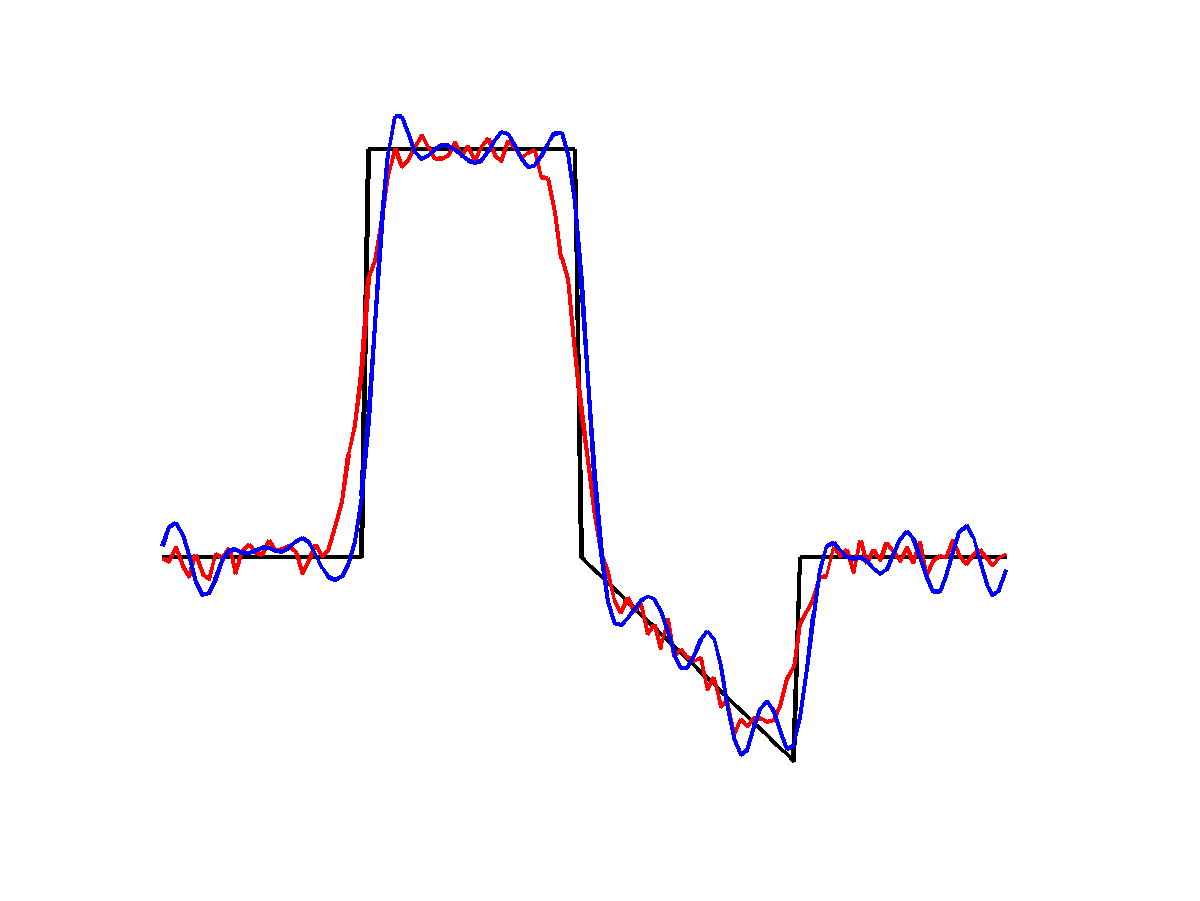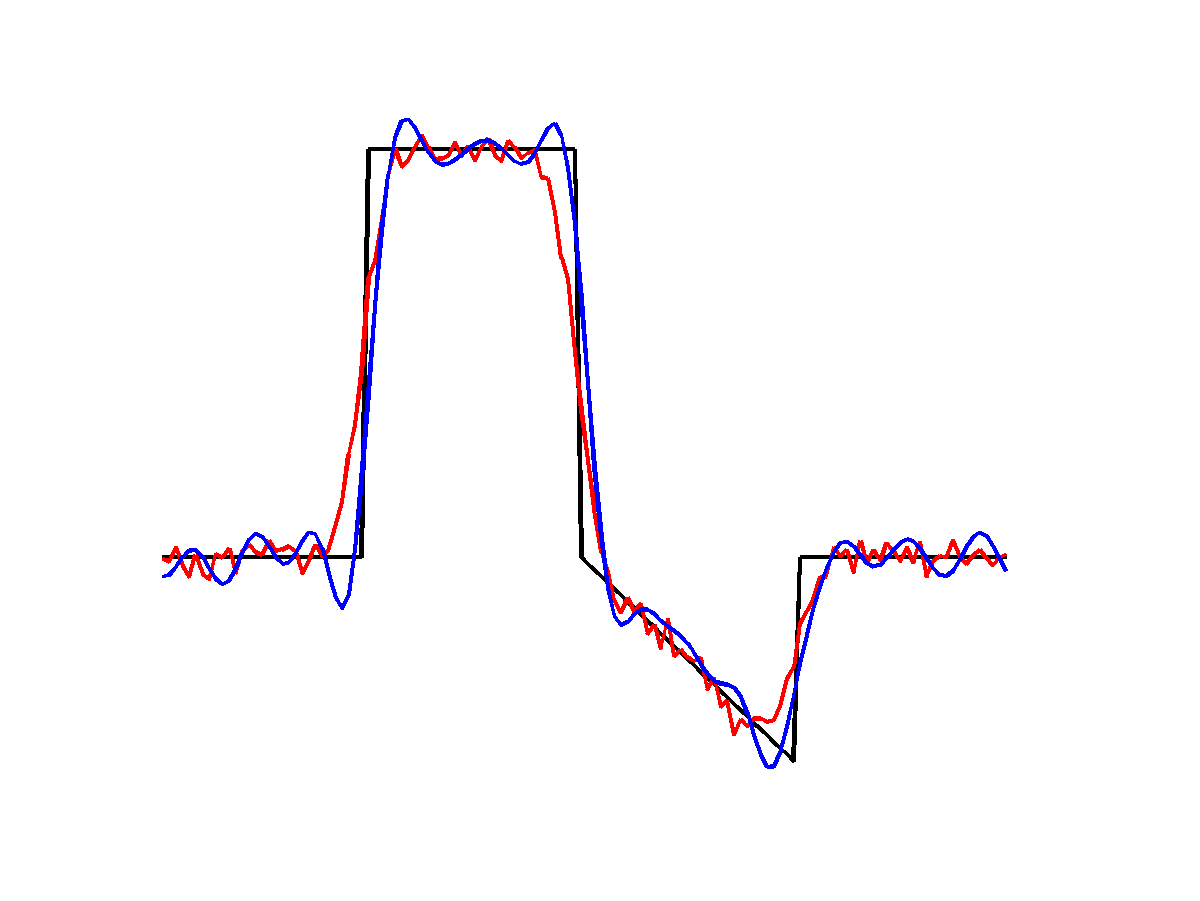## Sunday, November 29, 2015

### Flashing Pro-Micro Clone (atmega32u4) from Command Line

I recently got Sparkfun Pro Micro clone from Ebay.  In order to flash it with a hex file I had to quickly ground the reset pin twice and then run:

avrdude -v -patmega32u4 -cavr109 -P/dev/cu.usbmodem1411 -b57600 -D -Uflash:w:MassStorage.hex:i

## Wednesday, June 18, 2014

### A comparison of some deconvolution priors

Continuing with experiments based on ADMM I implemented and experimented with a few different deconvolution priors.  I chose several $\ell_2$ and $\ell_1$ priors based on sparse gradients, sparse curvature and simply the norm of the solution vector as well as an $\ell_{0.5}$ HyperLaplacian prior.

For all results the ground-truth signal is shown in black, the blurred and corrupted signal in red and the deblurred signal in blue.  As a ground-truth signal I'm using a simple piecewise linear signal with a slanted region, some constant regions and discontinuous jumps.  These features help to show the issues with different regularizers.  The input signal is 128 samples long, blurred by a Gaussian filter with $\sigma=4$ and then corrupted by Gaussian noise with $\sigma=2.5%$ and $\mu=0$.

For the $\ell_2$ results I'm solving for the optimal solution directly in the Fourier domain, while for the $\ell_1$ and $\ell_0.5$ I'm using ADMM, solving the data-subproblem in the Fourier domain and the splitting variable subproblem with shrinkage/proximal operators.  For all problems I've played with the prior weights a bit to try to get good results, but don't claim they are optimal.$\ell_2$ norm of solution vector
First up is the $\ell_2$ norm of the solution.  This does sharpen up the signal somewhat, but has bad ringing artifacts.  These are expected for the $\ell_2$ solution, particularly given the high noise levels in the corrupted signal.  The relatively large blur also leaves lots of freedom for low-frequency ringing.$\ell_2$ norm of solution first derivative
Results for the $\ell_2$-norm of the signal derivatives do a bit better, particularly for the slanted segment, but still show low-frequency ringing.  The same is true for the $\ell_2$-norm of the second derivative, although the ringing is a bit more pronounced in the flat regions:$\ell_2$ norm of solution second derivative
Moving on to the $\ell_1$ priors, the total-variation prior is simply the $1$-norm of the signal first derivatives. It favours piecewise constant solutions which do well on the large flat regions but introduce staircase artifacts for the slanted region:$\ell_1$ norm of solution first derivative
The total variation prior produces a much sharper signal than the $\ell_2$ priors, however for the slanted region it also introduces spurious features in the slanted region that are hard to distinguish from (correct) features elsewhere in the signal.  This occurs because the assumption of the total variation prior is that the input is piecewise constant, which is not true for this signal.  A better assumption is that the solution is piecewise linear, leading to the sparse-curvature prior.  The sparse-curvature assumes that the second derivative of the signal is non-zero in only a few locations:$\ell_1$ norm of solution second derivative
The sparse-curvature prior does much better in the slanted region than the total variation prior and still improves the signal, but the edges are not as sharp at discontinuities.  Often people combine these priors to try to achieve a compromise between the two behaviours.

The final prior is the Hyper-Laplacian prior, which is simply the $\ell_{0.5}$-norm of the signal first derivatives:$\ell_{0.5}$ norm of solution first derivative
The Hyper-Laplacian prior is non-convex, making it difficult to optimize for.  Here it appears that the optimization got stuck: some edges are extremely sharp, however the trailing edge of the high-amplitude constant region is lost entirely, possible because of the slanted region adjacent to it.  I played with the parameters quite a bit but did not get a result better than this.

Matlab example code generating these results is available at my GitHub repository:

https://github.com/jamesgregson/Matlab-deblurring-example

## Sunday, December 8, 2013

The title says it all, I've written some basic code for loading VTK rectilinear grid files into Matlab.  The code supports uniformly spaced meshes in up to four dimensions for both point and cell data.

https://github.com/jamesgregson/matlab_vtr

## Tuesday, October 22, 2013

### Index transformation between bounding boxes and uniform grids

I end up rewriting this code all the time. It transforms from points in space to grid coordinates world_to_grid() and back grid_to_world(), such that p = grid_to_world( aabb, dim, world_to_grid( aabb, dim, p ) ).  It's simple, but bugs here can mess up lots of things in ways that are hard to detect.  No range checking is performed in order to make it easy to apply custom boundary conditions.

    template< typename real, typename index, typename real3 >
inline real3 _world_to_grid( const real *aabb, const index *dim, const real3 &pos ){
return real3( real(dim-1)*(pos-aabb)/(aabb-aabb), real(dim-1)*(pos-aabb)/(aabb-aabb), real(dim-1)*(pos-aabb)/(aabb-aabb) );
}

template< typename real, typename index, typename real3 >
inline real3 _grid_to_world( const real *aabb, const index *dim, const real3 &pos ){
return real3( aabb+real(pos)*(aabb-aabb)/real(dim-1), aabb+real(pos)*(aabb-aabb)/real(dim-1), aabb+real(pos)*(aabb-aabb)/real(dim-1) );
}  

The code assumes that the dim variable holds the number of points in each direction (e.g. int dim[] = { nx, ny, nz };) and that the first point is coincident with the minimum value of the axis-aligned bounding box with the last point coincident with the maximum value of the bounding box. The aabb parameter is the axis-aligned bounding box defining the grid in world space, e.g. int aabb[] = { xmin, xmax, ymin, ymax, zmin, zmax };

## Thursday, October 10, 2013

### A follow-up to fluid simulation on non-uniform grids

In my last post, I discussed preliminary results for fluid simulation on non-uniform Cartesian grids.  In that post I showed some preliminary results, but there were some bugs that added disturbing artifacts.

I have fixed a number of bugs and now have a solver based on BFECC advection using min-max limited cubic interpolation for non-uniform and often highly anisotropic meshes for the velocity and pressure solve, with high-resolution uniform density fields for the density field.  The results are fairly impressive:
This image shows a volume rendering (in Paraview) of a simulation computed using the grid in the background.  Near the area of interest, the grid is uniform, but it grows very quickly (geometrically, with growth rate ~1.5) outside this region.  The velocity/pressure grid is 133x127x134, but covers nearly a 10x6x10 m cubic volume, with 2cm cells in the fine region.  The density field is 1x6x1 m with 1cm uniform resolution.
Being able to run different resolutions and gradings for the velocity and density fields is extremely helpful: fine fluid details distract from a poor velocity solution, and high-resolution densities help avoid diffusion in the physics.  The image above shows the density as resolved by the fluid grid. It is terrible.  However the density as resolved by the density grid is -way- better:

It's still not perfect, but given the cell size and anisotropy, I think it does extremely well.  Although there are definite artifacts, the payoff is in the memory usage and runtime. The whole simulation is 5 seconds in real time and takes approximately 22 seconds per output frame, meaning my 5 second simulation completes in under an hour.  These simulations used to take on the order of 4-5 hours.

The results look pretty good.  I think the grading is too steep to get really nice results, but it's an excellent proof-of-concept.

## Tuesday, October 8, 2013

### Fluid Simulation for Graphics on Non-Uniform Structured Grids

I've been playing around with fluid simulation on non-uniform structured grids.  They have some charms in that it is easy to have very large domains with isolated regions of interest; e.g. near the camera or salient fluid features.  One of the big advantages is that it makes far-field boundaries easy, you simply extend the mesh far away from the domain.

My solver pretty run-of-the-mill; only the pressure solver was updated in order to handle anisotropic cells.  I've used a finite-volume formulation to derive the pressure-correction equation for this case, but it suffices to say that only the weights used in forming the Poisson equation change.

Here is an example, a grid that is roughly 10x10x5 meters, with 2cm fine cells for roughly 100x100x100 cells:

The aspect ratios get quite high, 100:1 is not uncommon.   You can see a buoyant flow simulation that I'm running as the contour values.  The simulation takes about 20 seconds per frame, of which 7 seconds is writing the VTK output files that I use to post-process.  In spite of that, the visual detail in the region of interest is excellent (given the resolution):

I find that it helps to advect a uniform resolution density field that is roughly 2X the fine cell resolution.  Writing the code with general-purpose fields that can be point-sampled arbitrarily makes this a trivial feature to implement, simply allocate different grids for density and velocity.

Finally here is a rendering of the final simulation in Paraview, total simulation time ~35 minutes. There are still some bugs to track down, but the results are pretty promising:

## Sunday, September 8, 2013

### Updated C Mathematical Expression Parser

I've updated and posted my recursive descent mathematical expression parsing code.  The new code is available from my Github repository:

https://github.com/jamesgregson/expression_parser

The original post describing the library is at: http://jamesgregson.blogspot.ca/2012/06/mathematical-expression-parser-in-c.html

New features include Boolean operations and a callback interface for custom named variables and functions.  You can optionally disable the Boolean operations (and the 10 levels of parsing they trigger) if not needed.